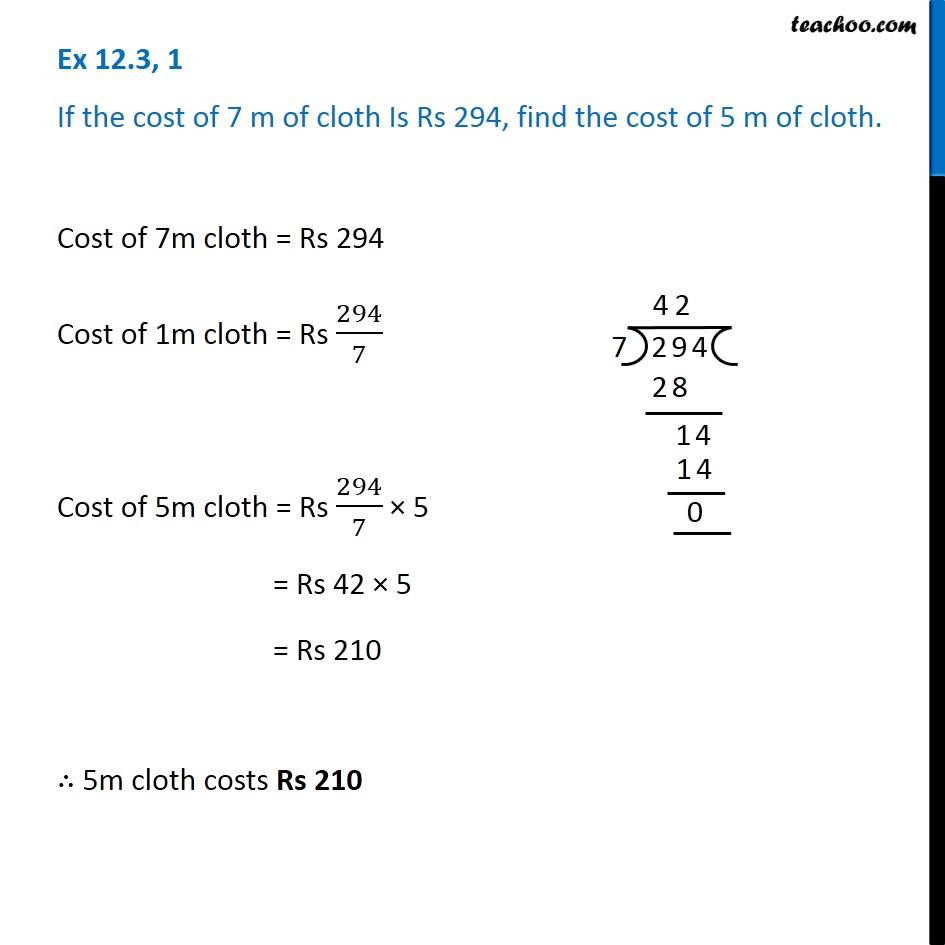Unitary Method

Chapter 12 Class 6 Ratio And Proportion
Concept wiseIntroducing your new favourite teacher - Teachoo Black, at only ₹83 per month

### Transcript

Ex 12.3, 1 If the cost of 7 m of cloth Is Rs 294, find the cost of 5 m of cloth.Cost of 7m cloth = Rs 294 Cost of 1m cloth = Rs 294/7 Cost of 5m cloth = Rs 294/7 × 5 = Rs 42 × 5 = Rs 210 ∴ 5m cloth costs Rs 210